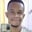Related Tags

javascript
communitycreator

# Loose equality on truthy and falsy values in JavaScriptChidume Nnamdi

In JavaScript, we have the Boolean value, which can exist in two states: true or false, or we can denote them with 1 and 0. Some values in JavaScript can either resolve to true or false. Values that resolve to true are called truthy values, and values that resolve to false are called falsy values.

In this shot, we will learn about the effect of the loose equality operator on truthy and falsy values in JavaScript.

### Loose equality on truthy and falsy values

Loose equality is the double = sign == . Loose equality is used to loosely check if two pieces of data are equal.

== coerces the data to a string before comparison, which results in strange results.

“0” is a truthy value. While 0 is a false value. Loose comparing tells us the values are equal.

console.log(0 == "0")
console.log("0" == 0)

true
true


== will convert 0 to “0” before comparing, and 0 == “0” will become “0” == “0”.

If the RHS is a number, == will convert it to a string and compare, so “0” == 0 will be “0” == “0”.

In loose equality, null and undefined are only true to themselves and nothing else.

console.log(null == undefined)
// true

console.log(undefined == null)
// true


The code above is a loose equality rule.

NaN is not equal to anything.

NaN == 0
// false

NaN == true
// false

NaN == false
// false

NaN == null
// false

NaN == undefined
// false


An empty array [] is equal to 0.

[] == 0
// true

[] == true
// false

[] == false
// true


RELATED TAGS

javascript
communitycreator

CONTRIBUTORChidume Nnamdi
RELATED COURSES

View all Courses

Keep Exploring

Learn in-demand tech skills in half the time